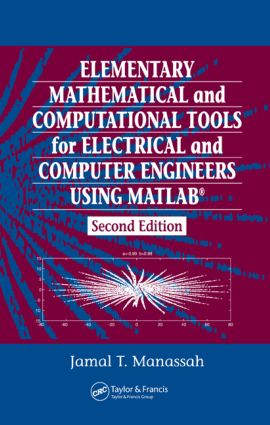# Elementary Mathematical and Computational Tools for Electrical and Computer Engineers Using MATLAB

## 2nd Edition

CRC Press

480 pages | 50 B/W Illus.

Hardback: 9780849374258
pub: 2006-10-20
\$125.00
x
eBook (VitalSource) : 9781315215747
pub: 2017-12-19
from \$62.50

FREE Standard Shipping!

### Description

Engineers around the world depend on MATLAB for its power, usability, and outstanding graphics capabilities. Yet too often, engineering students are either left on their own to acquire the background they need to use MATLAB, or they must learn the program concurrently within an advanced course. Both of these options delay students from solving realistic design problems, especially when they do not have a text focused on applications relevant to their field and written at the appropriate level of mathematics.

Ideal for use as a short-course textbook and for self-study Elementary Mathematical and Computational Tools for Electrical and Computer Engineers Using MATLAB fills that gap. Accessible after just one semester of calculus, it introduces the many practical analytical and numerical tools that are essential to success both in future studies and in professional life. Sharply focused on the needs of the electrical and computer engineering communities, the text provides a wealth of relevant exercises and design problems. Changes in MATLAB's version 6.0 are included in a special addendum.

The lack of skills in fundamental quantitative tools can seriously impede progress in one's engineering studies or career. By working through this text, either in a lecture/lab environment or by themselves, readers will not only begin mastering MATLAB, but they will also hone their analytical and computational skills to a level that will help them to enjoy and succeed in subsequent electrical and computer engineering pursuits.

### Reviews

"…Manassah got it just right: his book lays out standard topics for a one-semester course. He presents enough of the mathematics to motivate a method, explains how to use MATLAB, and then assigns typical problems as exercises."

- CHOICE, March 2002

INTRODUCTION TO MATLAB AND ITS GRAPHICS CAPABILITIES

Getting Started

Basic Algebraic Operations and Functions

Plotting Points

M-files

MATLAB Simple Programming

Array Operations

Curve and Surface Plotting

Polar Plots

Animation

Histograms

Printing and Saving Work in MATLAB

MATLAB Commands Review

DIFFERENCE EQUATIONS

Simple Linear Form

Amortization

An Iterative Geometric Construct: The Koch Curve

Solution of Linear Constant Coefficients Difference Equations

Convolution-Summation of First-Order System with Constant Coefficients

General First Order Linear Difference Equations

Nonlinear Difference Equations

Fractals and Computer Art

Generation of Special Functions from their Recursion Relations

ELEMENTARY FUNCTIONS AND SOME OF THEIR USES

Function Files

Examples with Affine Functions

Examples with Polynomial Functions

Examples with Trigonometric Functions

Examples with the Logarithmic Function

Examples with the Exponential Function

Examples with Hyperbolic Functions and their Inverses

Commonly Used Signal Processing Functions

Animation of a Moving Rectangular Pulse

MATLAB Commands Review

NUMERICAL DIFFERENTIATION, INTEGRATION, AND SOLUTIONS OF ORDINARY DIFFERENTIAL EQUATIONS

Limits of Indeterminate Forms

Derivative of a Function

Infinite Sums

Numerical Integration

A Better Numerical Differentiator

A Better Numerical Integrator: Simpson's Rule

Numerical Solution of ODE

MATLAB Commands Review

ROOT SOLVING AND OPTIMIZATION METHODS

Finding the Real Roots of a Function

Roots of a Polynomial

Optimization Methods

MATLAB Commands Review

COMPLEX NUMBERS

Introduction

The Basics

Complex Conjugation and Division

Polar Form of Complex Numbers

Analytical Solutions of Constant Coefficients ODE

Phasors

Interference and Diffraction of Electromagnetic Waves

Solving ac Circuits with Phasors